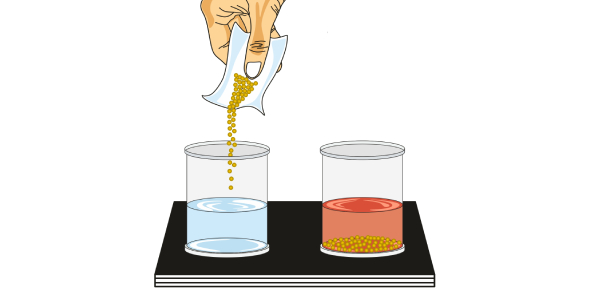# Chemical Equilibrium Class 11 MCQ Quiz With Answers

10 Questions | Total Attempts: 16SettingsIf you're studying in class 11 and looking for a chemical equilibrium MCQ quiz, then look nowhere else. Here, we have come up with an interesting quiz that consists of equilibrium questions and answers from the latest exam pattern. So, play it out and test your understanding. Chemical equilibrium is a state in a chemical reaction where no net change in the concentration of reactants and products occurs. How much more do you know? Learn more about it with the following quiz. Good luck!

• 1.
Reaction from which we can get back the reactants form products is called
• A.

Irreversible reaction

• B.

Reversible reaction

• C.

Endothermic reaction

• D.

Exothermic reaction

• 2.
The colour change when copper sulphate is heated is
• A.

Blue to white

• B.

White to blue

• C.

Blue to pink

• D.

Pink to blue

• 3.
Adding water to anhydrous copper sulphate is a ...............change
• A.

Endothermic

• B.

Exothermic

• C.

Both

• D.

None

• 4.
During equilibrium
• A.

Rate of forward reaction is more than backward reaction

• B.

Rate of backwards reaction is more than forward

• C.

Both forward and backward reaction has same rate

• D.

Both forward and backward reaction has different rate

• 5.
The amount of reactant and product remain .................during equilibrium
• A.

Equal

• B.

Constant

• C.

Different

• D.

Semi different

• 6.
The solubility of Ca3​(PO4​​)2​ in water is y mol/L. The solubility product will be
• A.

36 y4

• B.

6y²

• C.

108 y5

• D.

64 y5

• 7.
What is an acid according to Lewis concept?
• A.

Electron pair acceptor

• B.

Proton acceptor

• C.

Electron pair donor

• D.

Proton donor

• 8.
The pH of a solution of hydrochloric acid is 4. What is the molarity of the solution?
• A.

0.4

• B.

0.0001

• C.

0.04

• D.

4.0

• 9.
Strong conjugate base is out of these following:
• A.

Cl

• B.

NO32-

• C.

CH3COO

• D.

SO42-

• 10.
The weakest base out of the following is:
• A.

Ca(OH)2

• B.

KOH

• C.

NH4OH

• D.

NaOH

Related TopicsBack to top